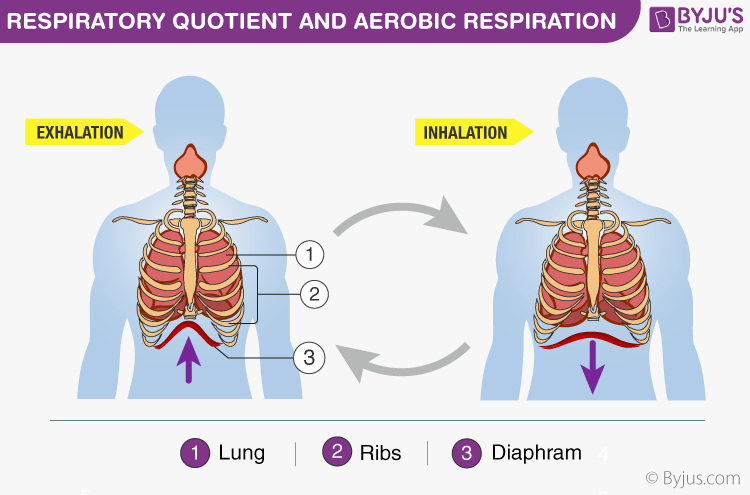# Respiratory Quotient And Aerobic Respiration

Respiration by definition describes it as the act of breathing; the inhalation and exhalation of gases are well familiar with each one of us. This time, we venture across a different aspect of it.

Explore more: Respiration

Let’s see what is the respiratory quotient and how it relates to aerobic respiration.## Respiratory Quotient

Aerobic respiration is the process most living things undergo to use food energy efficiently. In this aerobic respiration, oxygen is consumed and carbon dioxide is released.

The actual ratio of the volume of carbon dioxide eliminated to the volume of oxygen consumed during the act of cellular respiration is called the respiratory quotient. It is also referred to as the respiratory ratio. It is denoted by RQ.

Respiratory quotient may be represented as:

$$\begin{array}{l}RQ\end{array}$$
=
$$\begin{array}{l}\frac{Volume~ of ~Carbon~ dioxide~eliminated}{ Volume~ of~ Oxygen~ consumed}\end{array}$$

The respiratory quotient depends on the type of respiratory substrate used during the act of respiration. Additionally, the respiratory quotient is a dimensionless number used in the calculations of the basal metabolic rate or BMR when estimated from the carbon dioxide production. These measurements are forms of indirect calorimetry. It happens to be measured using Ganong’s respirometer.

When the carbohydrates are completely oxidized which are used as a substrate, the respiratory quotient becomes one. Here the equal amounts of carbon dioxide and oxygen are evolved and consumed, respectively.

$$\begin{array}{l}C_6H_{12}O_6 + 6 O_2 → 6 CO_2+ 6 H_2O + Energy\end{array}$$

$$\begin{array}{l}RQ\end{array}$$
=
$$\begin{array}{l}\frac{6 ~CO_2}{6 ~O_2}\end{array}$$

The RQ for carbohydrates is approximately 1.

But at the moment fats tend to involve in the act of cellular respiration, the respiratory quotient drops to lower than one.

For example,

$$\begin{array}{l}C_{16}H_{32}O_2 + 23 O_2 → 16 CO_2 + 16 H_2O\end{array}$$

$$\begin{array}{l}RQ\end{array}$$
=
$$\begin{array}{l}\frac{16~ CO_2}{23~ O2}\end{array}$$
=
$$\begin{array}{l}0.696\end{array}$$

The RQ for fats is approximately 0.7.

When proteins are respiratory substrates the ratio would be about 0.9. For example,

$$\begin{array}{l}C_{72}H_{112}N_{18}O_{22}S + 77 ~O_2 → 63~ CO_2 + 38~ H_2O + SO_3 + 9 ~CO (NH_2)_2\end{array}$$

$$\begin{array}{l}RQ\end{array}$$
=
$$\begin{array}{l}\frac{63 ~CO_2}{77 ~O_2}\end{array}$$
=
$$\begin{array}{l}0.8\end{array}$$

The RQ for protein is approximately 0.8.

One must recognize that in living organisms the respiratory quotient is rarely one, in fact, most often, it happens to be more than one. Pure proteins and fats are quite honestly never used as respiratory substrates.

## Application of Respiratory Quotient

Applications of the respiratory quotient can be found in severe cases of chronic obstructive pulmonary disease. Other applications include:

• Used as an indicator of over or underfeeding.
• Used for analysing the functioning of the liver.
• Used to predict weight gain in non-insulin-dependent diabetic patients.
• Used for diagnosing the patient’s conditions, who are suffering from liver cirrhosis and non-protein respiratory quotient.